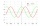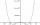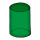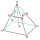#### Number of problems found: 17

• Substitution methodSolve goniometric equation: sin4 θ - 1/cos2 θ=cos2 θ - 2
• Hyperbola equationFind the hyperbola equation with the center of S [0; 0], passing through the points: A [5; 3] B [8; -10]By introducing a new variable solve biquadratic equation: ?
• Eq2 2Solve following equation with quadratic members and rational function: (x2+1)/(x-4) + (x2-1)/(x+3) = 23
• Sequences AP + GPThe three numbers that make up the arithmetic sequence have the sum of 30. If we subtract from the first 5, from the second 4 and keep the third, we get the geometric sequence. Find AP and GP members.
• Geometric progressiobIf the sum of four consective terms of geometric progression is 80 and arithmetic mean of second and fourth term is 30 then find terms?
• Three members GPThe sum of three numbers in GP (geometric progression) is 21 and the sum of their squares is 189. Find the numbers.
• CuboidCuboid with edge a=6 cm and space diagonal u=31 cm has volume V=900 cm3. Calculate the length of the other edges.
• Right triangle eq2Find the lengths of the sides and the angles in the right triangle. Given area S = 210 and perimeter o = 70.
• Diamond diagonalsFind the diamond diagonal's lengths if the area is 156 cm2 and side is 13 cm long.
• Rotary cylinderIn the rotary cylinder it is given: surface S = 96 cm2 and volume V = 192 cm cubic. Calculate its radius and height.
• The cylinderIn a rotating cylinder it is given: the surface of the shell (without bases) S = 96 cm2 and the volume V = 192 cm cubic. Calculate the radius and height of this cylinder.
• Faces diagonalsIf a cuboid's diagonals are x, y, and z (wall diagonals or three faces), then find the cuboid volume. Solve for x=1.3, y=1, z=1.2
• Two chordsCalculate the length of chord AB and perpendicular chord BC to circle if AB is 4 cm from the center of the circle and BC 8 cm from the center of the circle.
• Diamond diagonalsCalculate the diamonds' diagonals lengths if the diamond area is 156 cm square, and the side length is 13 cm.
• MO Z8-I-1 2018Fero and David meet daily in the elevator. One morning they found that if they multiply their current age, they get 238. If they did the same after four years, this product would be 378. Determine the sum of the current ages of Fero and David.
• Truncated pyramidThe truncated regular quadrilateral pyramid has a volume of 74 cm3, a height v = 6 cm, and an area of the lower base 15 cm2 greater than the upper base's content. Calculate the area of the upper base.

Do you have an interesting mathematical word problem that you can't solve it? Submit a math problem, and we can try to solve it.

We will send a solution to your e-mail address. Solved examples are also published here. Please enter the e-mail correctly and check whether you don't have a full mailbox.

Please do not submit problems from current active competitions such as Mathematical Olympiad, correspondence seminars etc...

Looking for help with calculating roots of a quadratic equation? Bikvadratic equation - math problems. Quadratic Equations Problems.